weixin_57972756 2021-11-02 11:55 采纳率: 50%浏览 1398

# 如何用python求解三角函数方程呢？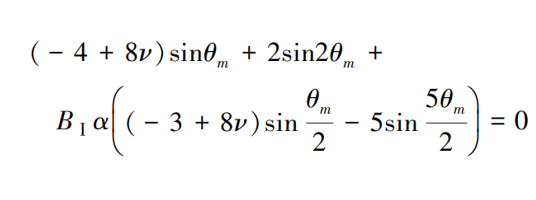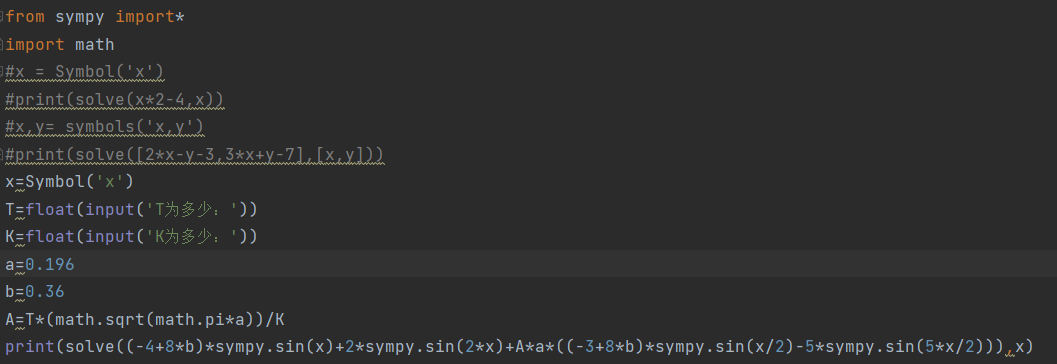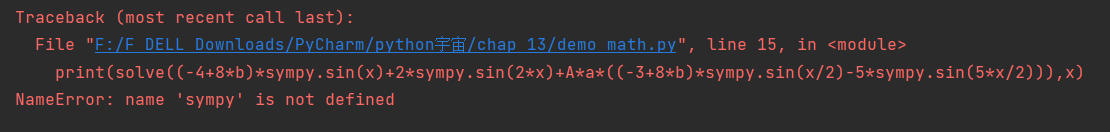``````from sympy import*
import math
x=Symbol('x')
T=float(input('T为多少：'))
K=float(input('K为多少：'))
a=0.196
b=0.36
A=T*(math.sqrt(math.pi*a))/K
print(solve((-4+8*b)*sin(x)+2*sin(2*x)+A*a*((-3+8*b)*sin(x/2)-5*sin(5*x/2))),x)
``````
• 写回答

#### 2条回答默认 最新

•「已注销」 2021-11-02 16:00
关注

math中的sin()函数有错误，用sympy的sin()函数；
另外，solve(fx,x)你的x的位置写到solve外面去了；
另外，我改好了以后发现程序也是执行不出来，拆解成两部分运行后，发现后半部分的式子解不出来，查了下资料可以是要nsolve解决
但是nsolve解出来的是近似值，精度应该也是很高的，但是，函数的x要换成0，代码在下面
另外，你的k和t被我写死了，自己改下哈~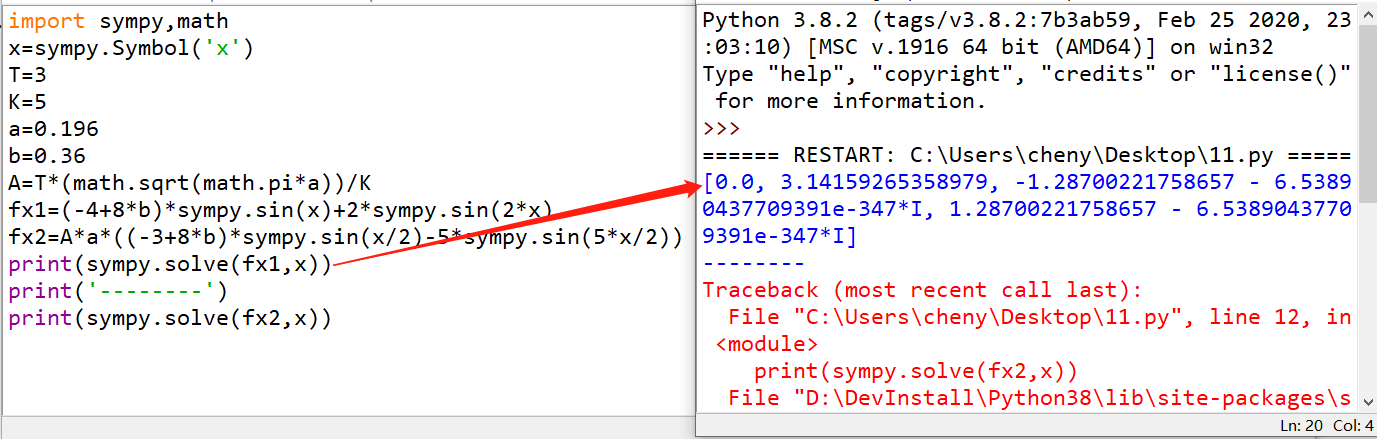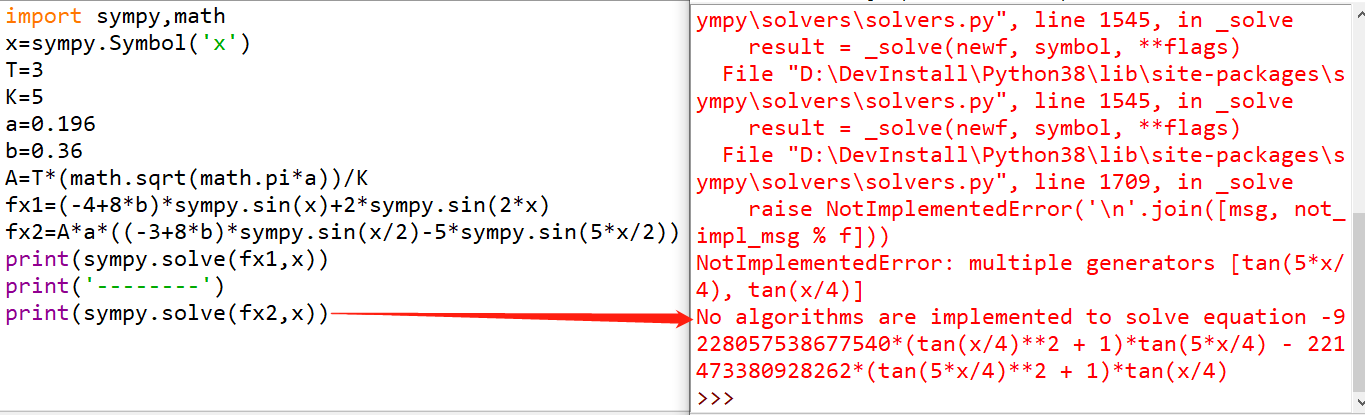``````import sympy,math
x=sympy.Symbol('x')
T=3
K=5
a=0.196
b=0.36
A=T*(math.sqrt(math.pi*a))/K
fx=(-4+8*b)*sympy.sin(x)+2*sympy.sin(2*x)+A*a*((-3+8*b)*sympy.sin(x/2)-5*sympy.sin(5*x/2))
print(sympy.nsolve(fx,0))
``````
本回答被题主选为最佳回答 , 对您是否有帮助呢?
评论

#### 悬赏问题

• ¥15 div editable中的光标问题
• ¥15 mysql报错1415Not allowed to return a result set from a trigger 不知如何修改
• ¥60 Python输出Excel数据整理，算法较为复杂
• ¥15 看一下这个，可以商量
• ¥15 回答几个问题 关于数据库
• ¥15 51单片机串口通信问题，未完成且要修改
• ¥15 百鸡问题 c++编程问题（相关搜索：输出数据）
• ¥30 如何在CMD中设置代理
• ¥15 我有一块薛定谔的硬盘
• ¥15 微信小游戏开发2D碰撞检测问题